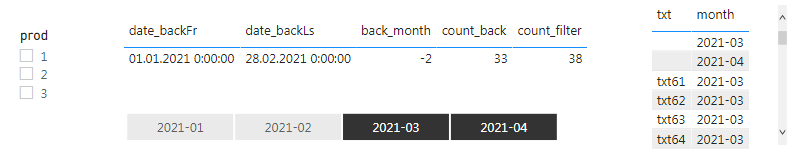cancel
Showing results for
Did you mean:Frequent Visitor

Hi All !

we have 2 simple datasets + Calendar:our screen:Our steps:

1. choice Products in slicer Prod or leave it unselected
2. choice month in slicer Month (one or more)
3. Measure [back_month] - contains the number of selected months

``back_month = (DATEDIFF(MIN('calendar'[Date]),MAX('calendar'[Date]),MONTH)+1)*-1``

4. Measure [count_filter] - contains the total number of filtered rows in the txt-table for the selected months (point 2) and Products (point 1)

5.  The problem in the [count_back].

Measure [count_back] - must containts the total number filtered rows in the txt-table for [back_month] months ago.

``````count_back =
VAR back_month = (DATEDIFF(MIN('calendar'[Date]),MAX('calendar'[Date]),MONTH)+1)*-1
RETURN count_back``````

You can see the expected date-intervals for filtering [count_back] in Measures [date_backFr] & [date_backLs]

``````date_backFr = FIRSTDATE(DATEADD('calendar'[Date],[back_month],MONTH))

For example,
if we chose the Month interval: (2021-03) & (2021-04), then in the Measure [count_back] we must calculated the number of rows in txt for the previous month-intervals (-2 months) => (2021-01) & (2021-02)

if we chose the Month interval: (2021-03), then in the Measure [count_back] we must calculated the number of rows in txt for the previous month-interval (-1 month) => (2021-02)

At now, the measure [count_back] work correctly only if we do not use the Prod filter.

If we turn Propuct filter is on, then everything breaks down ((
How to use additional filtering by Prod in this case?

Regards,

1 ACCEPTED SOLUTIONFrequent Visitor

I was modify my formula (using PARALLELPERIOD ) and it worked!

``````Nprev =
VAR monthBack = (DATEDIFF(MIN('rsp'[date]),MAX('rsp'[date]),MONTH)+1)*-1
VAR xStart = STARTOFMONTH(PARALLELPERIOD(rsp[date],monthBack,MONTH))
VAR xEnd = ENDOFMONTH(PARALLELPERIOD(rsp[date],monthBack,MONTH))
VAR cntx = CALCULATE(COUNTROWS(txt),FILTER(ALL(rsp[date],rsp[month]),rsp[date]>=xStart && rsp[date]<=xEnd))

RETURN cntx``````
3 REPLIES 3Super User

@StenX , Try like

same period based on date range
Last Period =
var _max =maxx(date,date[date])
var _min =maxx(date,date[date])
var datediff1 = datediff(_min,_max,day)
var _maxX = _max-datediff1
var _minX = _min -datediff1
return
CALCULATE(SUM(Sales[Sales Amount]),filter(all(date),date[date]<=_maxX &&date[date]>=_minX)))Frequent Visitor

Thank you for your quick response!
My apologies for the delay in reply. Our office was closed for the weekend ((

I applied your idea to calculate for a displacement of the full month(s).

``````count_MonthBack =
VAR xDiffMonthBack = (DATEDIFF(MIN('calendar'[Date]),MAX('calendar'[Date]),MONTH)+1)*-1
VAR xCountBack=CALCULATE([cnt],FILTER(all(rsp),rsp[date]>=xStartBack && rsp[date]<=xEndBack))
RETURN xCountBack``````

Unfortunately, I couldn't do it. If we use the slicer Prod, everything breaks.
Perhaps the problem is in the DATEADD(). How can this be fixed for month metrics?

For check:

if we select the month 2021-04, then the date of the week should be filtered by 01.03.2021-31.03.2021 (one month)

if we select the month 2021-04&2021-03, then the date of the week should be filtered by 01.01.2021-28.02.2021 (two months)

Regards,Frequent Visitor

I was modify my formula (using PARALLELPERIOD ) and it worked!

``````Nprev =
VAR monthBack = (DATEDIFF(MIN('rsp'[date]),MAX('rsp'[date]),MONTH)+1)*-1
VAR xStart = STARTOFMONTH(PARALLELPERIOD(rsp[date],monthBack,MONTH))
VAR xEnd = ENDOFMONTH(PARALLELPERIOD(rsp[date],monthBack,MONTH))
VAR cntx = CALCULATE(COUNTROWS(txt),FILTER(ALL(rsp[date],rsp[month]),rsp[date]>=xStart && rsp[date]<=xEnd))

RETURN cntx``````